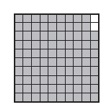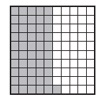# Finding Benchmark Fractions and Percentages for a Figure

#### Complete Python Prime Pack

9 Courses     2 eBooks

#### Artificial Intelligence & Machine Learning Prime Pack

6 Courses     1 eBooks

#### Java Prime Pack

9 Courses     2 eBooks

Sr.No Fraction Benchmark Percentage
1

$\frac{1}{10}$

10%
2

$\frac{1}{5}$

20%
3

$\frac{1}{4}$

25%
4

$\frac{1}{2}$

50%
5

$\frac{3}{4}$

75%
6 1 100%

What percent of the grid is shaded? Choose the closest benchmark percent and fraction.### Solution

Step 1:

We are given a 10 × 10 grid with 100 squares. The number of shaded squares gives the percentage of shaded squares as it is out 100 squares.

Step 2:

The number of shaded squares is 11. So, the percentage of shaded squares is 11%. This is estimated to nearest benchmark percentage 10%. The equivalent benchmark fraction is therefore $\frac{1}{10}$

What percent of the grid is shaded? Choose the closest benchmark percent.### Solution

Step 1:

We are given a 10 × 10 grid with 100 squares. The number of shaded squares gives the percentage of shaded squares as it is out 100 squares.

Step 2:

The number of shaded squares is 98. So, the percentage of shaded squares is 98%. This is estimated to nearest benchmark percentage 100%. The equivalent benchmark fraction is therefore 1.

What percent of the grid is shaded? Choose the closest benchmark percent.### Solution

Step 1:

We are given a 10 × 10 grid with 100 squares. The number of shaded squares gives the percentage of shaded squares as it is out 100 squares.

Step 2:

The number of shaded squares is 52. So, the percentage of shaded squares is 52%. This is estimated to nearest benchmark percentage 50%. The equivalent benchmark fraction is therefore $\frac{1}{2}$